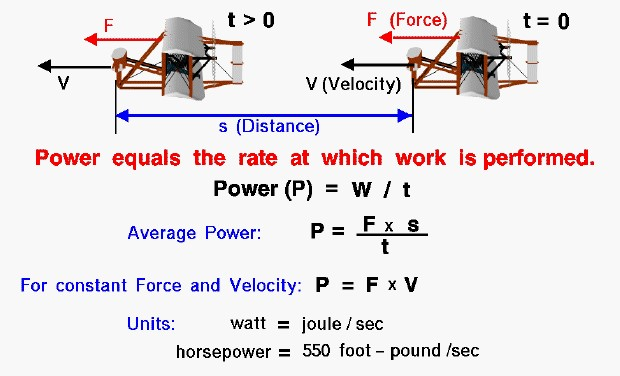# What is Power?For scientists, power is the rate at which work is performed by a force acting along a distance. As an example shown on the slide, the Wright 1903 aircraft is acted upon by the thrust force (F) from time (t) equals zero to some later time (t > 0) and travels some distance (s). The work (W) done on the aircraft during this time is F times s.

### W = F * s

The average power (P) expended by the engine to perform this work is equal to the work divided by the time.

### P = F * s / t

The unit of power in the metric system is the watt, which is equal to one joule per second. In the English system the unit of power is the horsepower (hp) which is equal to 550 foot-pounds per second. In our simple example, the force is a constant value aligned with the displacement of the aircraft and the velocity (V) is constant. The power then becomes the product of the force and the velocity:

### P = F * V

Let’s look closely at our example. If our aircraft developed 100 pounds of thrust and we flew 110 feet, it would require 11,000 foot-pounds of work. If we fly the distance in 10 seconds, we need to exert 1100 foot-pounds per second, or 2 horsepower. A perfectly efficient 2 horsepower engine would deliver enough power to generate 100 pounds of thrust at a velocity of 11 feet/sec. Suppose we increase the speed to 22 feet/sec. We would now cover the 110 feet distance in 5 seconds. The work would still be 11,000 foot-pounds (100 pounds of thrust for 110 feet). But the power required is now 2200 foot-pounds per second, or 4 horsepower. Increased horsepower lets you fly faster with the same amount of thrust, or it lets you generate more thrust at the same velocity.

## Calculating Power Requirements

The Wright brothers used their knowledge of work and power to determine the minimum requirements for their 1903 engine design. They were able to make rather accurate estimates of the drag of their 1903 aircraft based on the flight tests of the 1902 glider and the 1901 wind tunnel results. Knowing the drag and the desired flight velocity they computed the power requirements for the engine in order to develop thrust to overcome the drag of the aircraft.

Provide feedback